# Bihar Board Class 9th Maths Solutions Chapter 10 Circles Ex 10.6

Bihar Board Class 9th Maths Solutions Chapter 10 Circles Ex 10.6 Textbook Questions and Answers.

## BSEB Bihar Board Class 9th Maths Solutions Chapter 10 Circles Ex 10.6Question 1.
Prove that the line of centres of two intersecting circles subtends equal angles at the two points of intersection.
Solution:
Given : Two circles with centres A and B, which intersect each other at C and D.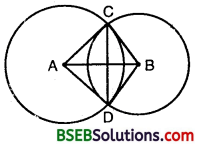To prove : ∠ACB = ∠ADB
Construction : Join AC, AD, BD and BC.
Proof: In ∆s ACB and ADB, we have
BC = BD [Radii of the same circle]
∴ By SSS criterion of congruence,

Question 2.
Two chords AB and CD of length 5 cm and 11 respectively of a circle are parallel to each other and are on opposite sides of its centre. If the distance between AB and CD is 6 cm, find the radius of the circle.
Solution:
Let O be the centre of the given circle and let its radius be r cm. Draw OP ⊥ AB and OQ ⊥ CD. Since OP ⊥ AB, OQ ⊥ CD and AB || CD. Therefore, points collinear. So, PQ = 6 cm.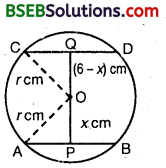Let OP = x. Then, OQ = (6 – x) cm.
Join OA and OC. Then, OA = OC – r.
Since the perpendicular from the centre to a chord of the circle bisects the chord.
∴ AP = PB = 2.5 cm and CQ = QD = 5.5 cm.
In right ∆s OAP and OCQ, we have
OA² = OP² + AP² and OC² = OQ² + CQ²
⇒ r² = x² + (2.5)² … (1)
and r² = (6 – x)² + (5.5)² … (2)
⇒ x² + (2.5)² = (6 – x)² + (5.5)²
⇒ x² + 6.25 = 36 – 12x + x² + 30.25
⇒ 12x = 60 ⇒ x = 5
Putting x = 5 in (1), we get
r² = 5² + (2.5)2 = 25 + 6.25 = 31.25
⇒ r = $$\sqrt{31.25}$$ = 5.6 (approx.)
Hence, the radius of the circle is 5.6 cm (approx.)Question 3.
The lengths of two parallel chords of a circle are 6 cm and 8 cm. If the smaller chord is at distance 4 cm from the centre, what is the distance of the other chord from the centre.
Solution:
Let AB and CD be two parallel chords of a circle with centre O such that AB = 6 cm and CD = 8 cm.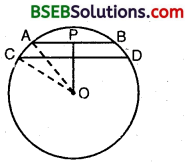Let the radius of the circle be r cm.
Draw OP ± AB and OQ ⊥ CD. Since AB || CD and OP ⊥ AB, OQ ⊥ CD.
Therefore, points O, Q a,nd P are collinear. Clearly OP = 4 cm, and P, Q are mid-points of AB and CD respectively.
AD = PB = $$\frac { 1 }{ 2 }$$ AB = 3cm
and, CQ = QD = $$\frac { 1 }{ 2 }$$ CD = 4 cm
In rt. ∠D A OAP, we have
OA² = OP² + AP²
⇒ r² = 4² + 3² = 16 + 9 = 25
⇒ r = 5
In rt. ∠D A OCQ, we have
OC² = OQ² + CQ²
⇒ r² = OQ² + 4²
⇒ 25 = OQ² + 16
⇒ OQ² = 9
⇒ OQ = 3
Hence, the distance of chord CD from the centre is 3‘ cm.

Question 4.
Let the vertex of an angle ABC be located outside a circle and let the sides of the angle intersect equal chords AD and CE with the circle. Prove that ∠ABC is equal to half the difference of the angles subtended by the chords AC and DE at the centre.
Solution:
Since an exterior angle of a triangle is equal to the sum of the interior opposite angles.In Δ BDC, we have ∠ADC = ∠DBC + ∠DCB…(1)
Since angle at the centre is twice the angle at a point on the remaining part of circle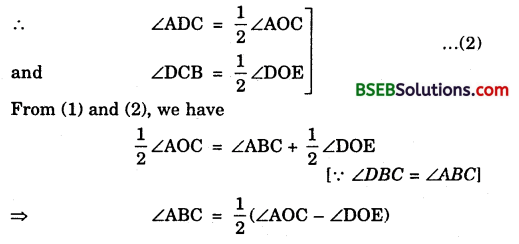Hence, ∠ABC is equal to half the difference of angles subtended by the chords AC and DE at the centre.Question 5.
Prove that the circle drawn with any side of a rhombus as diameter, passes through the point of intersection of its diagonals.
Solution:
Given : ABCD is a rhombus.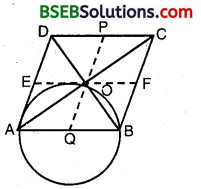AC and BD are its two diagonals which bisect each other at right angles.
To prove : A circle drawn on AB as diameter will pass through O.
Construction : From O draw PQ || AD and EF || AB.
Proof:
∴ AB = DC ⇒ $$\frac { 1 }{ 2 }$$ AB = $$\frac { 1 }{ 2 }$$ DC
⇒ AQ = DP [∵ Q and P are mid-points of AB and CD]
Similarly AE = OQ
⇒ AQ = OQ = QB
⇒ A circle drawn with Q as centre and radius AQ passes through A, O and B.
The circle thus obtained is the required circle,

Question 6.
ABCD is a parallelogram. The circle through A, B and C intersect CD (produced if necessary) at E. Prove that AE = AD.
Solution:
In order to prove that AE = AD i.e., A AED is an isosceles triangle it is sufficient to prove that ∠AED = ∠ADE.
Since ABCE is a cyclic quadrilateral.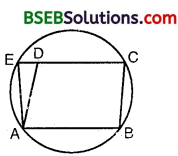∴ ∠AED + ∠ABC = 180° … (1)
Now, CDE is a straight line.
From (1) and (2), we get
∠AED + ∠ABC = ∠ADE + ∠ABC
∴ In A AED, we haveQuestion 7.
AC and BD are chords of a circle which bisect each other. Prove that (i) AC and BD are diameters, (ii) ABCD is a rectangle.
Solution:
(i) Let AB and CD be two chords of a circle with center 0.
Let they bisect each other at O.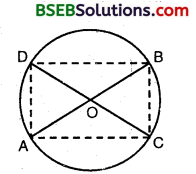Join AC, BD, AD and BC.
In As AOC and BOD, we have
OA = OB [∵ O is the mid-point of AB]
∠AOC = ∠BOD Wert. opp. ∠s]
and, OC = OD [∵ O is the mid-point of CD]
By SAS criterion of congruence,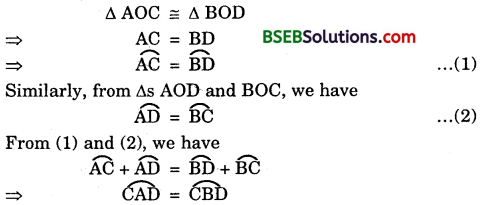⇒ CD divides the circle into two parts
⇒ CD is a diameter.
Similarly, AB is a diameter.

(ii) Since ∆ AOC ≅ ∆ BOD [Prove above]
⇒ ∠OAC i.e., ∠BAC = ∠OBD i.e., ∠ABD
AC || BD
Again, ∆ AOD ≅ ∆ COB
⇒ ACBD is a cyclic ||gm.
⇒ ∠DAC = ∠DBA … (3) [Opp. ∠s of a ||gm are equal]
Also, ACBD is a cyclic quadrilateral
∴ ∠DAC + ∠DBA = 180°
From (3) and (4), we get
∠DAC = ∠DBA = 90°
Hence, ABCD is a rectangle.Question 8.
Bisectors of angles A, B and C of a triangle ABC intersect its circumcircle at D, E and F respectively. Prove that the angles of the triangle DEF are 90° $$\frac { 1 }{ 2 }$$ A, 90° – $$\frac { 1 }{ 2 }$$ B and 90° – $$\frac { 1 }{ 2 }$$ C.
Solution:We have ∠D = ∠EDF
= ∠EBA + ∠FCA [∵∠ED A and ∠EBA are the angles in the same Segment of the circle]
∴ ∠EDA = ∠EBA. Similarly, ∠ADF and ∠FCA are the angles in the same segment and hence, ∠ADF = ∠FCA]
= $$\frac { 1 }{ 2 }$$∠B + $$\frac { 1 }{ 2 }$$∠C
[∵ BE is the internal bisector of ∠B and CF is the internal bisector ∠C]
= $$\frac{\angle \mathrm{B}+\angle \mathrm{C}}{2}$$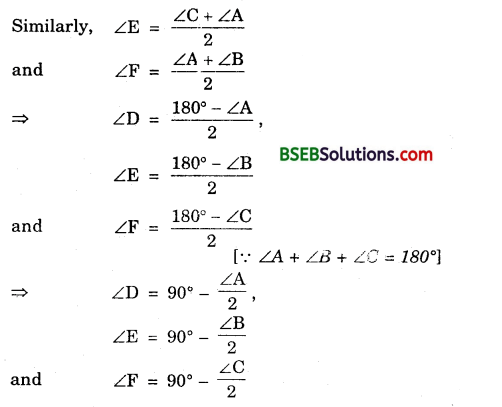⇒ The angles of the A DEF are 90° – $$\frac { 1 }{ 2 }$$A, 90° – $$\frac { 1 }{ 2 }$$B and 90° – $$\frac { 1 }{ 2 }$$C.

Question 9.
Two congruent circles intersect each other at points A and B. Through A any line segment PAQ is drawn so that P, Q lie on the two circles. Prove that BP = BQ.
Solution:
Let O and O’ be the centre of two congruent circles.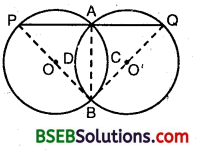Since AB is a common chqrd of these circles.
∴ arc ACB = arc ADB
⇒ ∠BPA = ∠BQA
⇒ BP = BQ.Question 10.
In any triangle ABC, if the angle bisector of ∠A and perpendicular bisector of BC intersect, prove that they intersect on the circumcircle of the triangle ABC.
Given : ABC is a triangle inscribed in a circle with centre at O. E is a point on the circle such that AE is the internal bisector of ∠BAC and D is the mid-point of BC.To prove : DE is the right bisector of BC i.e., ∠BDE = ∠CDE = 90°.
Construction : Join BE and EC.
Solution:
In ∆ BDE and ∆ CDE, we have BE = CE
BE = CE
[∵∠BAE = ∠CAE, ∴ arc BE = arc CE ⇒ chord BE = chord CE]
BD = CD [Given]
DE = DE [Common]
∴ By SSS criterion of congruence,
∆ BDE ≅ ∆ CDE
⇒ ∠BDE = ∠CDE [C.P.C.T]
Also, ∠BDE + ∠CDE = 180° [Liner Pair]
∴ ∠BDE = ∠CDE = 90°
Hence, DE is the right bisector of BC.